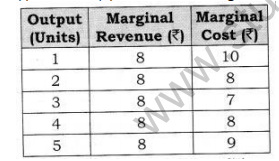# NCERT Solutions Class 12 Economics Producer Equilibrium

## Producer Equilibrium Class 12 NCERT Solutions

Class 12 Economics students should refer to the following NCERT questions with answers for Producer Equilibrium in standard 12. These NCERT Solutions with answers for Grade 12 Economics will come in exams and help you to score good marks

### Producer Equilibrium NCERT Solutions Class 12

NCERT Solutions Class 12 Economics Producer Equilibrium. NCERT book for Economics in class 12 is strongly recommened by teachers and The NCERT solutions for class 12 Economics free in PDF made by teachers of the best schools in India. These solutions are carefully compiled to give detailed understanding of the concepts and also steps of solutions. The NCERT solutions are free to download in pdf format. Please refer to the download link below to download the pdf file and also refer to other chapters and subjects to get the solutions to Economics NCERT book questions and exercises.

Question 1. What conditions must hold if a profit- maximizing firm produces positive output in a competitive market?
Or  Explain the producer’s equilibrium with MR/MC approach (when Price remains constant with the rise in output). Or
Explain the conditions of a producer’s equilibrium in terms of marginal cost and marginal revenue. Use diagram [CBSE Sample Paper 2012, 13] Or
Why is the equality between marginal cost and marginal revenue necessary for a firm to be in equilibrium? Is it sufficient to ensure equilibrium? Explain. [CBSE 2015} Or Elaborate the implication of the conditions of equilibrium of a firm. [CBSE Sample Paper 2016]
Answer: The conditions must hold if a profit maximizing firm produces positive output in a competitive market when price is constant under MR/MC approach is determined where,
(i) MR = MC (ii) MC must be risingAccording to Table, both the conditions of equilibrium are satisfied at 4 units of output. MC is equal to MR and MC is rising. MC is more than MR when output is produced after 4 units of output. So, Producer’s Equilibrium will be achieved at 4 units of output. However, MR is equal to MC at 2 units of output also. But, second condition is not fulfilled here.
Let us understand the determination of equilibrium with the help of a diagram:
Producer’s Equilibrium is determined at OQ level of output corresponding to point E as at

## Books recommended by teachers

 NCERT Solutions Class 12 Economics Introduction to Economics
 NCERT Solutions Class 12 Economics Demand NCERT Solutions Class 12 Economics Elasticity of Demand
 NCERT Solutions Class 12 Economics Cost NCERT Solutions Class 12 Economics Production
 NCERT Solutions Class 12 Economics Perfect Competition NCERT Solutions Class 12 Economics Producer Equilibrium NCERT Solutions Class 12 Economics Revenue NCERT Solutions Class 12 Economics Supply
 NCERT Solutions Class 12 Economics Consumer Equilibrium NCERT Solutions Class 12 Economics Excess Demand and Deficient Demand NCERT Solutions Class 12 Economics Market Equilibrium with Simple Applications
 NCERT Solutions Class 12 Economics Non-Competitive Market
 NCERT Solutions Class 12 Economics Introduction to Macroeconomics and its Concepts
 NCERT Solutions Class 12 Economics National Income and Related Aggregates
 NCERT Solutions Class 12 Economics Banking NCERT Solutions Class 12 Economics Money
 NCERT Solutions Class 12 Economics Aggregate Demand and Its Related Concepts NCERT Solutions Class 12 Economics National Income Determination and Multiplier
 NCERT Solutions Class 12 Economics Government Budget and the Economy
 NCERT Solutions Class 12 Economics Balance of Payment NCERT Solutions Class 12 Economics Foreign Exchange Rate